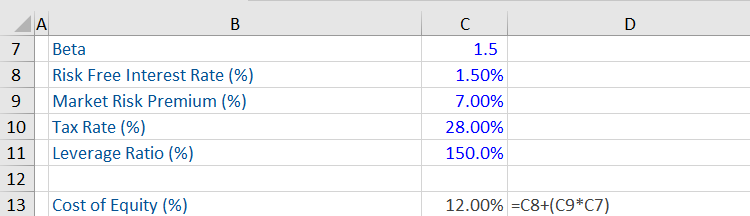## What is the “Capital Asset Pricing Model?”

The Capital Asset Pricing Model (CAPM) shows the relationship between the required or expected return on equity and risk of investing in the same. It is integral to calculating the weighted average cost of capital WACC (which is used extensively in financial modeling), as it computes the cost of equity and is important for the purpose of valuation in corporate finance.

## Key Learning Points

• The CAPM uses a risk free-rate, risk premium, and beta of an investment to calculate the cost of equity; and
• The CAPM calculates the cost of equity which has a very important application in the calculation of the WACC.

## CAPM, Cost of Equity, Beta and WACC

Stated below is the CAPM Model, which is used the calculate the cost of equity.

Ke = Rf + Bp * (Erm – Rf)

Ke = Required or expected return on equity or cost of capital (this is the return that is required or expected by shareholders)

Rf = Risk-free rate

Bp = Beta of an investment

Erm = relates to expected return in the market

(Erm – Rf) = Risk Premium for taking on equity risk

The term Rf refers to the return that investors require for taking no risk i.e. investing in something riskless. These are typically 10-year benchmark government bonds (for example, the 10-year US treasury bond) and the return or yield on such a security is the risk-free rate.

Next, we need to add on the risk premium for taking on equity risk ( (Rm – Rf) i.e. for investing in risky assets such as equities. Basically, the risk premium is the excess return on risky assets over and above the risk-free rate (on risk-free assets). Since investors invest in risky assets too, they need to obtain a return (i.e. compensation) that is higher or above the risk-free rate. The Erm can be based on historic or implied returns in the market, typically it is the long run historic average market return (e.g. 30 year average return for the S&P 500).

The beta of a stock/portfolio measures the systematic risk (i.e. market risks that cannot be diversified) and the sensitivity of a stock or portfolio relative to changes in the market. It indicates how volatile the stocks of a company are relative to the overall stock market It measures the stock risks relative to the overall market, if the beta of a stock is greater (less) than 1, this implies a greater (lower) level of risk and volatility compared to the market. For more information please see the following article on beta.

As CAPM is used to calculate the cost of equity, this forms a very important part of the WACC calculation. For more information please see the following article on WACC.

## CAPM, Cost of Equity, Leveraging Beta and WACC

In this example, there is a CAPM workout that uses the CAPM formula stated above to calculate the cost of equity (12%).